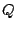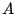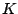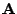Next: Robust statistics and yet Up: Estimating camera response function Previous: Smoothness and monotonicity constraints

### Computational efficiency

The least squares formulation of (13) can be solved using the standard approach for the normal equations. Debevecdebevec presented a similar least squares formuation, but his was much more computationally intensive because he introduced extra unnecessary variables corresponding to ourfor each exposure. Due to his large number of unnecessary variables he was forced to use a small number of hand-selected points to calculate a computationally tractable solution.

In contrast, our method (and earlier methods such as that of  ) is computationally tractable, such that it uses the information at all the pixels and hence could be expected to be much more immune to noise.

Our method is also fully automatic and does not require any hand selection of image portions in order to make it computationally tractable.

The method presented in this paper can be be made more efficient (typically hundreds of times more efficient than it already is) by the following:

• Recognizing that many rows ofare repeated, these rows can be grouped together into a single row and weighted by the square root of the number of repetitions. By weightingalso by the same factor, the same numerical solution results.
• Rows that are zero, which happen when the corresponding pixel location in both images has the same value, may be eliminated, further reducing the size of the matrix.Next: Robust statistics and yet Up: Estimating camera response function Previous: Smoothness and monotonicity constraints
Steve Mann 2002-05-25